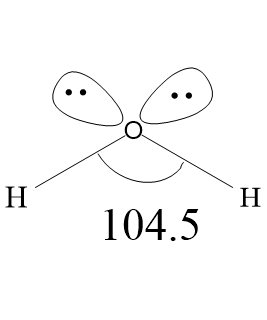QuestionAnswers

# Draw the shape of a water molecule.Verified
Hint: There will be two lone pairs and two bond pairs arranged around the central atom,and the oxygen atom will be $s{{p}^{3}}$ hybridized .
Water is a polar molecule with one oxygen atom bonded to two different hydrogen atoms. Due to high electronegativity of oxygen atoms, the covalent bonds formed between oxygen and hydrogen is polar. Oxygen atom acquires partial two partial negative charges (${{\delta }^{-}}{{\delta }^{-}}$) and each hydrogen atom have one partial positive charge (${{\delta }^{+}}$). Oxygen has two lone pairs of electrons. Therefore, the water molecule has two bond pairs and two lone pairs, arranged in tetrahedral geometry around the central atom oxygen. Generally the normal tetrahedral bond angle is ${{109.5}^{\circ }}$, but in water molecule, because of two lone pair- lone pair repulsion and lone pair-bond pair repulsion, the bond angle is decreased from ${{109.5}^{\circ }}$ to ${{104.5}^{\circ }}$. Leaving the lone pairs, the water molecules have bent shape. The oxygen atom acquires $s{{p}^{3}}$ hybridization. Because of the polarity of bonds, water molecules have high dipole moments, with negative charge directed towards the lone pair. Polar molecules attract one another by dipole dipole forces, as the negative part of one water molecule attracts the positive part of another water molecule. The hydrogen atom of water molecules has low electron density, therefore, the hydrogen atom of one molecule is attracted to the lone pair of the oxygen atom of another molecule.The bond length between Oxygen and Hydrogen atoms is 0.96${{A}^{\circ }}$. Vibrational normal modes are located at 3756 $c{{m}^{-1}}$ (symmetric stretch), 3657 $c{{m}^{-1}}$ (antisymmetric stretch), and 1595 $c{{m}^{-1}}$ (bend). Antisymmetric stretch is greater than symmetric stretch, due to greater change in dipole moment.